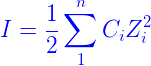# Ionic Strength Formula

The amount of ion concentration in the solution is the ionic strength of the solution. It is articulated as I. The ion activity is affected by it. It is denoted with the ion interaction with water and other ions in the solution. To compute the half of the total concentration of each ionic species, the ionic strength formula is used.
Ionic strength formula is articulated asCi – ionic concentration

Zi – ion charges

## Ionic Strength Examples

Problem 1: Calculate the ionic strength of KCl if its concentration is 2 M.

Given:

 Ions Z C Z2 K+ 1 2 1 Cl– 1 2 1

The ionic strength is given by
I =

$$\begin{array}{l}\frac{1}{2}\end{array}$$
[(2
$$\begin{array}{l}\times\end{array}$$
1) + (2
$$\begin{array}{l}\times\end{array}$$
1)]

= 2

Problem 2: Calculate the ionic strength of K2SO4.

Given

 Ions Z C Z2 K+ 1 2 1 SO4-2 2 1 4

The ionic strength is given by
I =

$$\begin{array}{l}\frac{1}{2}\end{array}$$
[(2
$$\begin{array}{l}\times\end{array}$$
1) + (1
$$\begin{array}{l}\times\end{array}$$
4)] = 3

 More topics in Ionic Strength Formula Net Ionic Formula Ionization Energy Formula